## Wednesday, November 3, 2021

### Field Waves: UNIZOR.COM - Physics4Teens - Waves

Notes to a video lecture on http://www.unizor.com

Field Waves

So far, our understanding of waves was very much related to a concept of some material object to oscillate, that is some material particles going back and forth, up and down, left and right or, in short, making some repetitive movements.

But Physics deals not only with material in a usual sense objects. A very important part of what physicists deal with is fields.

Let's spend a few minutes to talk about a nature of the concept field.

In Mathematics it's customary to analyze the properties of some mathematical objects (like N-dimensional vector space) without really having any real life object that corresponds exactly to this mathematical concept.

Mathematicians care only about properties, regardless of the objects that possess these properties. Moreover, there might be completely different mathematical objects that possess exactly the same properties, and the beauty of Mathematics allow us not to think about objects that possess these properties, but to concentrate only on analysis of the properties themselves.

With properly analyzed properties, mathematicians can apply the results to any mathematical object that possess these properties.
In short, properties are of primary interest, not objects that possess these properties.

With fields physicists do the same. They analyze the properties of the fields using some experiments, building a model that corresponds to these experiments and analyzing the properties of the model.

If at certain time they come up with a new experiment that contradicts the model, they change (enhance, update) the model and continue analyzing the model.

We don't really know what fields really are, but we can observe, experiment with, measure their properties, and, as long as our experiments do not contradict the results of our theoretical analysis, we accept the results of the theory.

So, for our purposes we consider a field as some area of space that has certain properties. In particular, we can experiment with some material objects and see how they behave inside these space areas we call fields, and that's how we observe the fields' properties.

The primary subject of analysis in this chapter is an electromagnetic field.
Our analysis will be based on material presented in the previous part of this course called "Electromagnetism".

The purpose of this lecture is to explain that electromagnetic field can oscillate and propagate even without any "material" (in a usual sense) carrier, allowing the light from stars to reach us through Space vacuum.

Let's start from the familiar fact that around a line of moving electrons (electric current) there exists a magnetic field.
If, for example, we take a straight wire and connect it to a direct current (DC) battery, the permanent flow of electrons through this wire will create constant magnetic field around it with concentric magnetic field lines.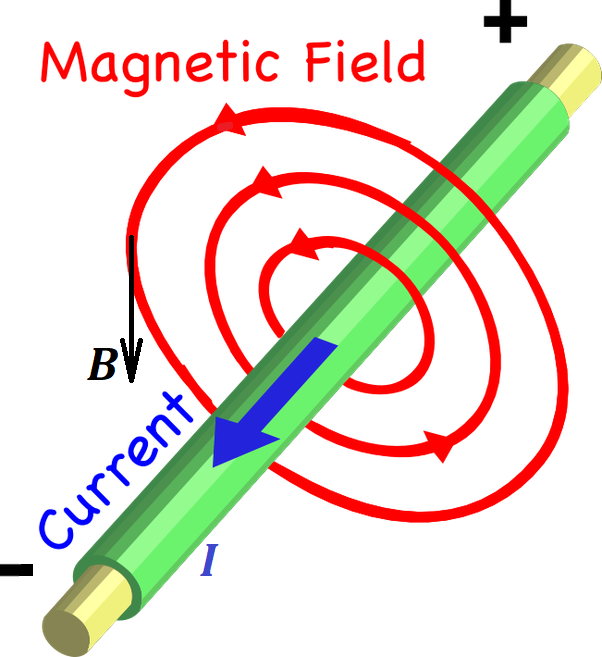The fact that electrons are moving signifies the existence of the electric field of some intensity, and the appearance of a magnetic field we attribute to properties of electric field.

The next logical step is that around a line of moving electrons, when the movement is not constant, but variable, there exists a variable magnetic field.
For example, if we have a wire loop and connect it to alternate current (AC) source of electricity that always changes the direction certain number of times per second, the magnetic field going through this wire loop also will change the direction of its magnetic field lines with each cycle of an electric current.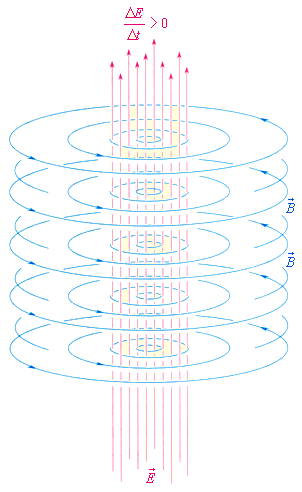The variability of the flow of electrons is caused by a variable electric field, and the appearance of a variable magnetic field we attribute to variability of an electric field.

The lecture "Straight Line Current" of this course (follow the menu items UNIZOR.COM - "Physics 4 Teens" - "Electromagnetism" - "Magnetism of Electric Current") explains this process in details for DC.
The lecture "Induction and AC" of this course (follow the menu items UNIZOR.COM - "Physics 4 Teens" - "Electromagnetism" - "Alternating Current Induction") explains this process in details for AC.

Our next experimental fact is the electromagnetic induction - a variable magnetic field around an electric wire causes appearance of a variable electric current in the wire that can only be caused by a variable electric field.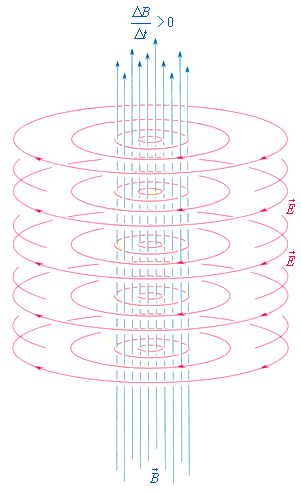So, we can conclude that a variable electric field causes the existence of a variable magnetic field, while a variable magnetic field causes the existence of a variable electric field.

Combining everything we mentioned above, we see that a variable electromagnetic field (a field where variable electric and magnetic forces exist) that consists of logically and physically chained together variable electric field and variable magnetic field, mutually causing each other, is self-sustained and capable of propagating through vacuum without existence of any wires as a medium.
This chain can schematically be represented as follows.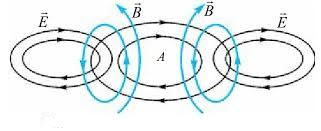Here a variable (oscillating) electric field causes the existence of a variable (oscillating) magnetic field in its neighborhood. That magnetic field causes the existence of another variable electric field further in space. This process repeats itself, thus facilitating the propagation of electromagnetic oscillations of an electromagnetic field.

Any field has certain forces acting on certain objects (or other fields).
The electromagnetic field at any point can be characterized by two forces - intensity vector of electric component of a field (E) and intensity vector of its magnetic component (B).

Here are a few facts about electromagnetic fields that require deeper immersion into their structure and are beyond the scope of this course.

1. Propagation of electromagnetic waves (called radiation in many cases) is perpendicular to both intensity vectors, electric and magnetic.
That makes these waves transverse.

2. Electric and magnetic intensity vectors are sinusoidal, perpendicular to each other and are in phase. Combining that property with their perpendicularity to a direction of propagation prompts the following graphical representation of electromagnetic waves.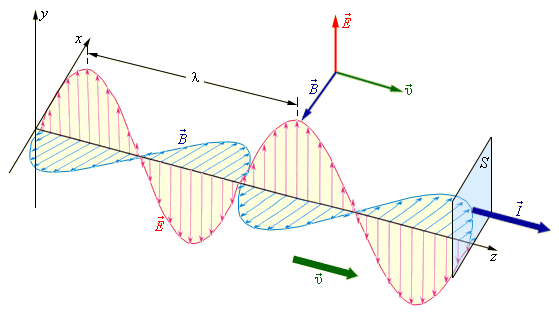(you can click the right mouse button and open this picture in another tab for better view)

3. Speed of propagation depends only on the properties of environment where the radiation is taking place.
In particular, the ability of electric field to permeate an environment (ε) and the ability of magnetic field to permeate an environment (μ) determine this speed.
The electric permittivity of vacuum is
ε0 ≅ 8.8542·10−12
.
The magnetic permeability of vacuum is
μ0 ≅ 1.2566·10−6
(H/m - Henry per meter)
.
Speed of electromagnetic waves c can be expressed in terms of electric permittivity and magnetic permeability as
c² = 1/(ε0·μ0)
According to the formula above and values of electric and magnetic constants, in vacuum the speed of electromagnetic waves is
c = 299,792,458 (m/sec)
which in many cases is rounded to 300,000 km/sec.